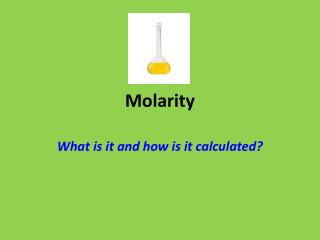Download PresentationMolarity

# Molarity - PowerPoint PPT Presentation

Molarity. What is it and how is it calculated?. Molarity. What is it?. A measurement of a solution’s concentration. How much solute is dissolved in a solvent. Moles of solute per Liter of solvent. m ol / L = M. Molarity. How is it calculated?. Moles ÷ Liter. g  mol.I am the owner, or an agent authorized to act on behalf of the owner, of the copyrighted work described.
Download Presentation## Molarity

Download Policy: Content on the Website is provided to you AS IS for your information and personal use and may not be sold / licensed / shared on other websites without getting consent from its author.While downloading, if for some reason you are not able to download a presentation, the publisher may have deleted the file from their server.

- - - - - - - - - - - - - - - - - - - - - - - - - - E N D - - - - - - - - - - - - - - - - - - - - - - - - - -
Presentation Transcript
1. Molarity What is it and how is it calculated?

2. Molarity What is it? • A measurement of a solution’s concentration • How much soluteis dissolved in a solvent • Moles of solute perLiter of solvent • mol / L = M

3. Molarity How is it calculated? • Moles ÷ Liter • g  mol • mL L

4. Representative Particles Molarity 1 mol______ 6.02 X 1023 particles ÷ L 6.02 X 1023 particles 1 mol x L Moles A Mol B Mol A atomic/molar mass from periodic table 1 mol Moles B Mol A Mol B _____1 mol_____ atomic/molar mass from periodic table Mass (grams)

5. Mole  Molarity # of moles x 1 = M L Example: Calculate the molarity if 0.450 gof Cr(NO3)2is dissolved in 500 mLof water. 0.450 gCr(NO3)2 x 1 molCr(NO3)2 = 2.56 x 10-3 mol 176 gCr(NO3)2 Cr(NO3)2 500 mLx 1 L= .5 L 103mL

6. Mole  Molarity 2.56 x 10-3 mol x 1 = 5 x 10-3 M Cr(NO3)2 .5 L

7. Mole  Molarity # of moles x 1 = M L Example: Calculate the molarity if 6.75 gof Na3 PO4is dissolved in 1000 mLof water. 4 x 10-1 MNa3PO4

8. Molarity Mole M x L = # of moles Example: Calculate the mass of AlCl3that was dissolved in 250 mLof water to make a 3.0 M solution. 250 mLx 1 L= .25 L 103mL .25 L x 3.0 molAlCl3 = 0.75 mol AlCl3 L

9. Molarity Mole 0.75 mol AlCl3x 132 g AlCl3 = 99 g AlCl3 1 molAlCl3

10. Molarity Mole M x L = # of moles Example: Calculate the mass of (NH4)2SO4that was dissolved in 750 mLof water to make a 2.5 M solution. 250g(NH4)2SO4